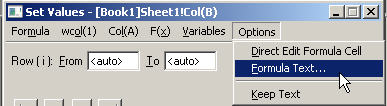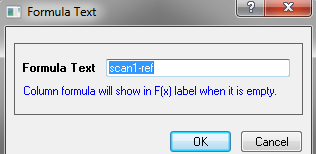# Column Formula Label Row

## Entering Column Formula on F(x) Row

You may notice when you first start Origin 9.1, that  a new label row named F(x) has been added to the default worksheet. This label row will allow you to directly enter and edit formula for setting column values in the column. The calculation will be set to recalculate automatically by default.For example:

2. Double-click in the F(x) cell of Column A, type
i
then press TAB to go to column B F(x) cell, and enter
sin(col(a))
then press Enter. Column B will be computed based on values in column A, and the recalculate mode will be set to Auto.

Right click workbook title and choose View: F(x) to turn on/off the formula label row. Then type formula directly using the same syntax as used in Set Values dialog. E.g. col(B)-col(A), sin(col(A)), i*2, etc.

You can use Set Values dialog as before to set formula. The formula label row will update.

## Support Explanatory Text for the Formula

Under Options menu in Set Values dialog, you can uncheck Direct edit Formula Cell. Then typing formula in F(x) row will not work anymore. User has to use Set Values dialog to set formula.

Then user can use Options: Formula Text... to enter some explanatory text for the formula, which will be displayed on F(x) row.